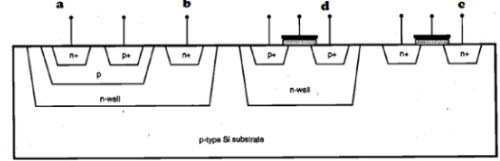Courses

# Test: BiCMOS Logic Gates

## 15 Questions MCQ Test VLSI System Design | Test: BiCMOS Logic Gates

Description
This mock test of Test: BiCMOS Logic Gates for Electrical Engineering (EE) helps you for every Electrical Engineering (EE) entrance exam. This contains 15 Multiple Choice Questions for Electrical Engineering (EE) Test: BiCMOS Logic Gates (mcq) to study with solutions a complete question bank. The solved questions answers in this Test: BiCMOS Logic Gates quiz give you a good mix of easy questions and tough questions. Electrical Engineering (EE) students definitely take this Test: BiCMOS Logic Gates exercise for a better result in the exam. You can find other Test: BiCMOS Logic Gates extra questions, long questions & short questions for Electrical Engineering (EE) on EduRev as well by searching above.
QUESTION: 1

### The BiCMOS are preferred over CMOS due to:

Solution:

These are the 3 advantages of BiCMOS over CMOS.

QUESTION: 2

### The transistors used in BiCMOS are:

Solution:

BiCMOS is a combination of both MOSFET and BJT.

QUESTION: 3

### The high current driving capability of the BiCMOS is due to:

Solution:

BJT has the high current driving capability.

QUESTION: 4

In BiCMOS inverter, the BJT used are:

Solution:

npn BJTs are used in BiCMOS inverter.

QUESTION: 5

The drawback of the BiCMOS circuits are:

Solution:

The other 2 are the merits of BiCMOS, Increased fabrication Complexity is a demerit of BiCMOS circuits.

QUESTION: 6

The Bipolar Transistor is fabricated on :

Solution:

BiCMOS is fabricated on the same substrate of nMOS.

QUESTION: 7

The n-well created for Bipolar Transistor in BiCMOS is used as:

Solution:

The created nWell is used as Collector region for BiCMOS.

QUESTION: 8

The n-well collector is formed by:

Solution:

To make the doping concentration less than the emitter.

QUESTION: 9

The collector contact region is doped with higher concentration of n-type impurities due to:

Solution:

The collector contact region is doped with higher concentration of n-type impurities reduces contact resistance.

QUESTION: 10

The proper BiCMOS inverter circuit is:

Solution:

None.

QUESTION: 11

In the above diagram of BiCMOS, the labels a,b,c,d denote:Solution:

None.

QUESTION: 12

The BJT in BiCMOS works as a:

Solution:

The Current Ic and Ie are controlled by base emitter bias voltage.

QUESTION: 13

In BiCMOS, the analysis of the operation of BJT is well explained by:

Solution:

None.

QUESTION: 14

The Ebers Moll equivalent circuit of BJT operating in forward active region is:

Solution:

None.

QUESTION: 15

The transfer characteristics of BiCMOS inverter is:

Solution:

None.